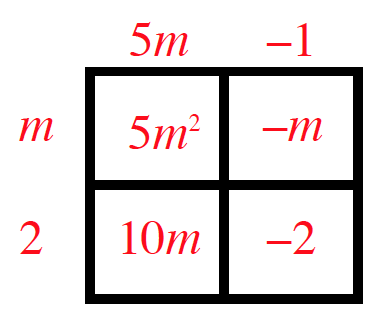Home > INT3 > Chapter 1 > Lesson 1.1.1 > Problem1-10

1-10.

Multiply each expression. Homework Help ✎

1. $(5m-1)(m+2)$

Use a rectangular area model.$5m^2+9m−2$

1. $(6-x)(2+x)$

Refer to part (a).

1. $(5x-y)^2$

Refer to part (a).

1. $3x(2x-5y+4)$

Refer to part (a).